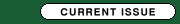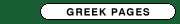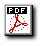Presentation of a regression equation to predict the Glasgow verbal score from the Glasgow eye and best motor score in patients with traumatic brain injury - a pilot study
MATIS G. ΜD MSc1, BIRBILIS T. MD PhD1, LYRATZOPOULOS N. MD PhD2, MINOPOULOS G. MD PhD2, MANOLAS K. MD PhD2
1Department of Neurosurgery - Democritus University of Thrace (DUTH), University General Hospital of Alexandroupolis, Greece
21st Department of Surgery - Democritus University of Thrace (DUTH), University General Hospital of Alexandroupolis, Greece

The Glasgow Coma Scale (GCS) represents an international model of recording neurological findings, comparing patients and predicting outcome. It is the sum of three components, namely the best motor response, the best verbal response, and eye opening. However calculating its verbal component in specific patients' subgroups (intoxication, medications, dementia, psychiatric diseases, developmental impairments, intubation, tracheostomy, laryngectomy, edema of tongue, facial trauma, mutism, hearing impairments) is extremely difficult. The aim of this pilot study was to derive a regression equation to accurately predict the verbal score based on the eye and motor scores. The data from 74 patients with traumatic brain injury who were admitted to the Intensive Care Unit (ICU) of University Hospital of Alexandroupolis, Greece (a tertiary care university hospital) during the decade 1994-2003 were obtained. The mean age and APACHE-II score was 45.19±2.555 and 15.91±0.890 respectively with the majority of the patients being male (87.8%) and living in an urban environment (58.1%). The main causes of injury were car accidents (75.7%) and falls (23%) and subjects were mainly conservatively treated (88.3%). The mean ICU length of stay was 11.5±2.051 days. 61.67% of patients presented a GCS score of 3 (all of whom were diagnosed with intraventricular hemorrhage), 10% a GCS score of 15 and 6.67% a GCS score of 14. With the aid of the statistical package SPSS (Statistical Package for Social Sciences) v. 14.0, the stepwise regression method was performed and the following equation was obtained: GCS-Verbal = 0.963 + 0.029*GCS-Eye*(GCS-Motor)2. This equation presented an adjusted R2 value of 0.916, a standard error of estimate value of 0.46 and a F value of 795.509, p=0.000. Correlation between actual and predicted verbal and total scores was calculated using the Pearson correlation coefficient and the Spearman rank correlation coefficient. The same question was addressed for other 3 regression equations which were proposed by other authors: (a) GCS-Verbal = -0.3756 + GCS-Motor*0.5713 + GCS-Eye*0.4233, (b) GCS-Verbal = 2.3976 + GCS-Motor*(-0.9253) + GCS-Eye*(-0.9214) + (GCS-Motor)2*0.2208 + (GCS-Eye)2*0.2318, and (c) GCS-Verbal = 0.2095 + GCS-Motor*(-2.1238) + GCS-Eye*3.9897 + (GCS-Motor)2*0.6048 + (GCS-Eye)2* (-1.9077) + (GCS-Motor)2*(-0.0344) + (GCS-Eye)3*0.2748. The discriminative ability of all 4 equations was tested using the Receiver Operating Characteristic curves (ROC). Various GCS scores (depending on the equation employed) were defined as test variables and outcome was defined as the state variable. The mean actual verbal and total Glasgow coma score was 1.9865±0.1861 and 6.3919±0.554 respectively. In the derived equation the corresponding mean values were 1.9865±0.178 and 6.3919±0.551 (r=0.958, rs=0.916, p=0.000 and r=0.995, rs=0.998, p=0.000). For the other 3 equations the mean predicted verbal score was 1.8732±0.195 (r=0.922, rs=0.920, p=0.000), 1.8726±0.160 (r=0.950, rs=0.924, p=0.000) and 1.9147±0.162 (r=0.951, rs=0.923, p=0.000), while the mean predicted total score was 6.2786±0.572 (r=0.991, rs=0.999, p=0.000), 6.2780±0.531 (r=0.995, rs=0.999, p=0.000) and 6.3201±0.534 (r=0.995, rs=0.999, p=0.000). The area under the ROC curves of total Glasgow coma scores for predicting survival was 0.545±0.076 (p=0.547) for the derived equation and 0.543±0.076 (p=0.567) for the other three equations. The corresponding value for actual ROC curve was 0.548±0.076 (p=0.523). The analysis of results demonstrates that the proposed equation predicts with great accuracy the verbal component in patients with traumatic brain injury, produces similar results with other regression equations brought forward by other authors and is simpler and less time consuming to solve. As drawbacks of this pilot study one should mention the relatively small sample size, the large number of patients with a GCS score of 3 (61.67%), the small number of patients with a GCS score within the intermediate values of this scale (where the mathematical relationship among its constituents is not so obvious and the predictive ability is more limited), and the lack of data collectors' experience (residents). However, the study is still in progress; it is our scope to include more than 200 patients with GCS scores other than 3. Final results will be available within 12 months. Encephalos 2009, 46(3):138-146.

Key words: Equations, Glasgow Coma Scale, regression, traumatic brain injury.FULL TEXT